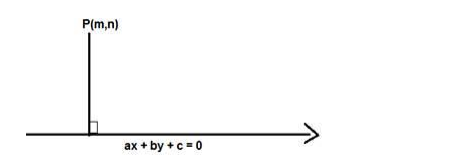# Find the distance of the point`
Question:

Find the distance of the point $(-4,3)$ from the line $4(x+5)=3(y-6)$

Solution:

Given: Point $(-4,3)$ and line $4(x+5)=3(y-6)$

To find: The distance of the point $(-4,3)$ from the line $4(x+5)=3(y-6)$

Formula used: We know that the distance between a point $P(m, n)$ and a line ax $+b y+$ $c=0$ is given by,

$D=\frac{|\mathrm{am}+\mathrm{bn}+\mathrm{c}|}{\sqrt{\mathrm{a}^{2}+\mathrm{b}^{2}}}$The equation of the line is $4 x+20=3 y-18$

$4 x-3 y+38=0$

Here $m=-4$ and $n=3, a=4, b=-3, c=38$

$D=\frac{|4(-4)-3(3)+38|}{\sqrt{4^{2}+3^{2}}}$

$D=\frac{|-16-9+38|}{\sqrt{16+9}}=\frac{|-25+38|}{\sqrt{25}}=\frac{|13|}{5}$

$D=\frac{13}{5}$

The distance of the point $(-4,3)$ from the line $4(x+5)=3(y-6)$ is $\frac{13}{5}$ units